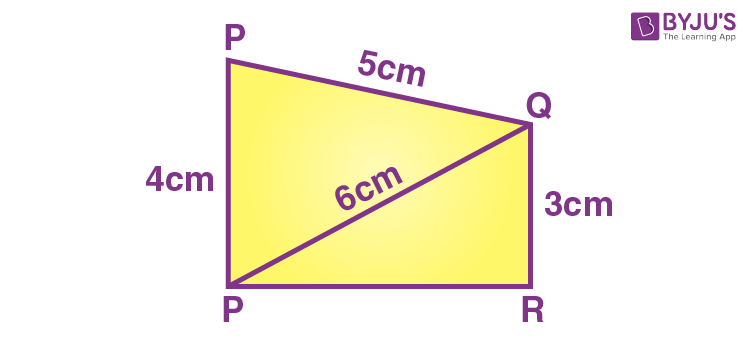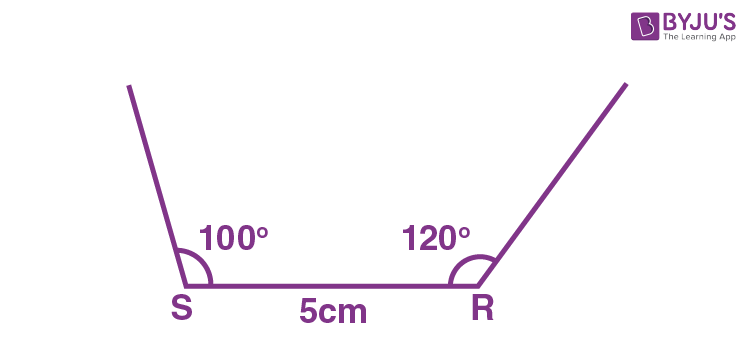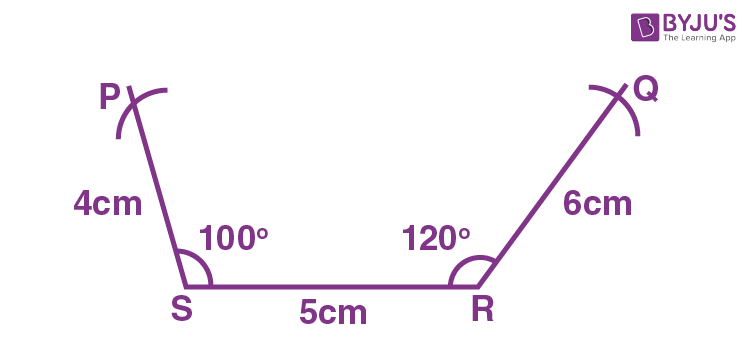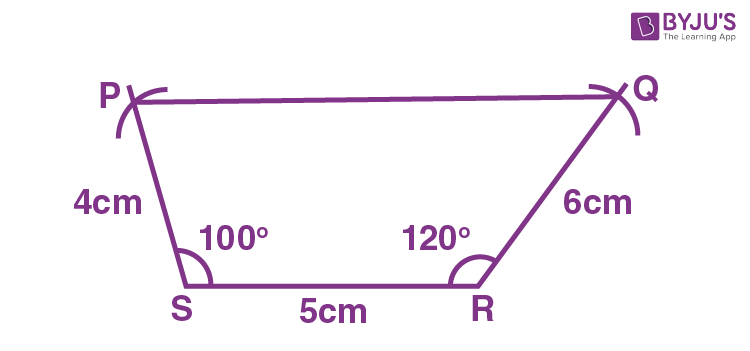# Constructing a quadrilateral with three sides and two angles.

Let us say you are required to construct a quadrilateral PQRS where the measurements are:

• QR = 6 cm
• RS = 5 cm
• PS = 4 cm
• ∠S = 100 degrees
• ∠R = 120 degrees

For the construction of quadrilaterals with some of the measurements given, we first draw a rough figure of the quadrilateral with the given dimensions, as shown below.Now starting with the construction, the steps are:

Step 1: Draw a line segment of length 5 cm and mark the ends as S and R.Step 2: Using a protractor, draw a line from point R making 120 degrees and another line from point S making 100 degrees with the line segment SR.Step 3: Set your compass to the radius of 4 cm and make an arc from the point S on the 100-degree line. Mark the point as P where the arc intersects the line.

Step 4: Similarly, set the compass to the radius of 6 cm and make an arc from point R on the 120-degree line. Mark the point as Q where the arc intersects the line.Step 5: Join the points P and Q.You obtain the quadrilateral PQRS of the required measurements.